### 2. THE COSMOLOGICAL CONSTANT AND VACUUM ENERGY

Vacuum fluctuations contributing togenerate a very large (formally infinite) value of the cosmological constant <T00>vac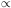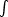0[k2 + m2]1/2 k2dk. The integral diverges as k4 resulting in an infinite value for <T00>vac and hence also for the cosmological constant= 8G <T00>vac. Since each form of energy gravitates and therefore reacts back on the space-time geometry, an infinite value ofis expected to generate an infinitely large space-time curvature through the semi-classical Einstein equations G00 = 8G / c4 <T00>vac. One way to avoid this is to assume that the Planck scale provides a natural ultra-violet cutoff to all field theoretic processes, this results in <T00>vac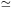c5 / G2~~ 1076GeV4 which is 123 orders of magnitude larger than the currently observed value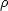10-47GeV4. A cutoff at the much lower QCD scale doesn't fare much better since it generates a cosmological constantQCD4 ~ 10-3GeV4 - forty orders of magnitude larger than observed. Clearly the answer to the cosmological constant issue must lie elsewhere.

The discovery of supersymmetry in the 1970's led to the hope that the cosmological constant problem may be resolved by a judicious balance between bosons and fermions in nature, since bosons and fermions (of identical mass) contribute equally but with opposite sign to the vacuum expectation value of physical quantities, so that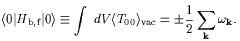(1)

However supersymmetry (if it exists) is broken at the low temperatures prevailing in the universe today and on this account one should expect the cosmological constant to vanish in the early universe, but to reappear during late times when the temperature has dropped below TSUSY. This is clearly an undesirable scenario and almost the very opposite of what one is looking for, since, a large value ofat an early time is useful from the viewpoint of inflation, whereas a very small current value ofis in agreement with observations.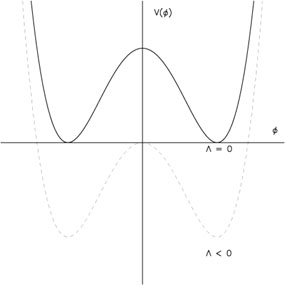Figure 1. The potential describing spontaneous symmetry breaking has the form of a Mexican top hat. The dashed line shows the potential before the cosmological constant has been `renormalized' and the solid line after.

The cosmological constant also makes an important appearance in models with spontaneous symmetry breaking . For simplicity consider the Lagrangian(2)

The symmetric state at= 0 is unstable and the system settles in the ground state= +or= -, where= [µ2 /]1/2, thus breaking the reflection symmetry-present in the Lagrangian. If V0 = 0 then this potential results in a broken symmetry state with a large negative cosmological constanteff = V(=) = - µ4 / 4. In order to avoid this situation the value of the free parameter V0 is chosen to counterbalanceeff, as a result one sets V0 ~ µ4 / 4so thateff / 8G = V0 - µ4 / 410-47GeV 4. The ensuing `regularization' of the large negative cosmological constant must be done with considerable care, since even small `fluctuations' in the final value ofcan result in grave consequences for cosmology. For instance ifeff / 8G < - 10-43GeV 4 the large attractive force exerted by a negative cosmological constant will ensure that the universe re-collapses before it reaches `maturity'. The age of the universe in this case will be < 1 billion years, much too short for galaxies to form and for life (as we know it) to emerge within the standard big bang scenario. On the other hand ifeff / 8G > 10-43GeV4, the large repulsive force generated bywill ensure that the universe begins accelerating before gravitationally bound systems have a chance to form. Such a scenario will also clearly preclude the emergence of life.

The rather small window permitted for life to emerge in the presence ofhas led several researchers [18, 19, 20] to develop anthropic arguments for the existence of a small cosmological constant. A possibility which is summarised by the following sentence: "if our big bang is just one of many big bangs, with a wide range of vacuum energies, then it is natural that some of these big bangs should have a vacuum energy in the narrow range where galaxies can form, and of course it is just these big bangs in which there could be astronomers and physicists wondering about the vacuum energy" . Anthropic arguments forwill not be examined further by me in this talk.

In the absence of a fundamental symmetry of nature which will set the value ofto zero one has to look towards physical mechanisms which might generate an acceptably small value of the-term today.

Exploring the connection between quantum fluctuations andZel'dovich suggested that, after the removal of divergences, the energy density of a virtual particle-antiparticle pair interacting gravitationally would be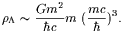(3)

(This result is easy to derive if one notes that the interaction energy density is typicallyvacvac c2 ~ (Gm2 /) /3 where=/ mc is the mean separation between particle and antiparticle.) This possibility has not been explored much, perhaps because the proton-antiproton (electron-positron) contribution gives a very large (small) value for. Interestingly the pion-antipion mass gives just the right value= 1 / (2)4Pl (m/ MPl)61.3 × 10-123Pl = 6.91 × 10-30 g cm-3 .

Purely numerological considerations also allow one to generate a sufficiently small value ofthrough a suitable combination of fundamental constants. For instance the fine structre constantcan be combined with the Planck densityPl to give=PL / (22)3 e-2/1.2 × 10-123Pl = 6.29 × 10-30 g cm-3.

A small vacuum energy may be connected to fundamental physics in other (equally speculative) ways. It is interesting that the mass scale associated with the scale of supersymmetry breaking in some models, MSUSY ~ 1 TeV, lies midway between the Planck scale and 10-3 eV. The small observed value of the cosmological constant(10-3eV)4 might therefore be associated with the vacuum in a theory which had a fundamental mass scale MXMSUSY2 / MPl, such thatvac ~ MX4 ~ (10-3eV)4.